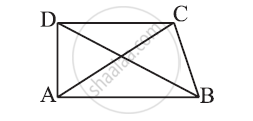Share

# Abcd is Quadrilateral. is Ab + Bc + Cd + Da >Ac + Bd? - CBSE Class 7 - Mathematics

ConceptSum of the Lengths of Two Sides of a Triangle

#### Question

Is AB + BC + CD + DA >AC + BD?#### Solution

In a triangle, the sum of the lengths of either two sides is always greater than the third side.

Considering ΔABC,

AB + BC > CA (i)

In ΔBCD,

BC + CD > DB (ii)

In ΔCDA,

CD + DA > AC (iii)

In ΔDAB,

DA + AB > DB (iv)

Adding equations (i), (ii), (iii), and (iv), we obtain

AB + BC + BC + CD + CD + DA + DA + AB > AC + BD + AC + BD

2AB + 2BC + 2CD +2DA > 2AC + 2BD

2(AB + BC + CD + DA) > 2(AC + BD)

(AB + BC + CD + DA) > (AC + BD)

Yes, the given expression is true.

Is there an error in this question or solution?

#### APPEARS IN

NCERT Solution for Mathematics for Class 7 (2018 to Current)
Chapter 6: The Triangle and its Properties
Ex. 6.40 | Q: 4 | Page no. 126
Solution Abcd is Quadrilateral. is Ab + Bc + Cd + Da >Ac + Bd? Concept: Sum of the Lengths of Two Sides of a Triangle.
S